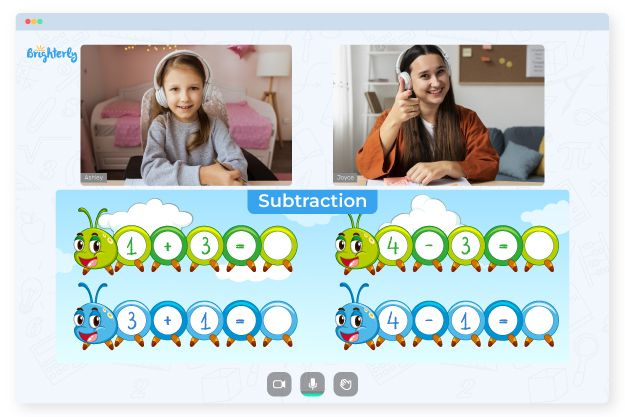# Subtraction With Regrouping Worksheets

Subtraction appears to be more challenging to kids than addition, just like division is more complex than multiplication. If you add regrouping to the equation, you will find that many young minds have no idea what to do with borrowed numbers.

To fill the knowledge gaps, teachers and parents use extra learning materials like worksheets. If you want to help your child master subtraction with regrouping without tears, you can get printable subtraction-with-regrouping worksheets at Brighterly. These assets consist of diversified math drills designed to solidify students’ knowledge of the topic.

## Three Benefits of Subtraction-with-Regrouping Worksheets

Check three advantages of using math worksheets at home:

### Subtraction-with-Regrouping Worksheets Enhance Computational Accuracy

It’s okay for students to make errors as they learn new topics. But it’s not the case on the math test. The good news is that you can give your child colorful interactive math worksheets to practice subtraction with regrouping to enhance their calculation accuracy.

With enough practice, students will borrow numbers correctly in their minds, and no math tests will scare them anymore.

### Subtraction-with-Regrouping Worksheets Solidify Acquired Math Skills

Some kids will still struggle with regrouping large numbers even if they succeed in subtracting smaller ones. And what about fractions? These are even more perplexing to young minds. Kids just need to practice different math strategies a bit more. This is when a subtracting fractions-with-regrouping worksheet will come in handy.

Math for Kids

Is Your Child Struggling With Math?
1:1 Online Math TutoringBrighterly’s worksheets contain diversified math exercises that foster children to apply different strategies and learn the ropes of this topic to the fullest.

### Subtraction Worksheets with Regrouping Increase Calculation Speed

Your little student may perform calculations correctly, but the process may take a long time. If children spend so much time doing homework while sacrificing their free time, they will quickly lose interest in math. In this case, students need more practice to refine their skills with worksheets.### Subtraction With Regrouping Worksheets PDF

Subtraction Worksheets With Regrouping### Subtraction With Regrouping Worksheets PDF

Subtraction Regrouping Worksheets### Subtraction With Regrouping Worksheets PDF

Regroup Subtraction Worksheets### Subtraction With Regrouping Worksheets PDF

Subtraction With Regrouping Worksheet

If students regroup in subtraction with worksheets long enough, they will solve similar equations much faster than at the beginning of learning the topic.

## Get Free Printable Subtraction-with-Regrouping Worksheets in PDF

Brighterly understands how frustrating it can be to google for educational materials on the web. After all, many websites provide only paid assets and require time-consuming steps to get them. At Brighterly, you can reduce all the hassle into a few clicks – select a subtraction-with-regrouping worksheet in PDF, download it, and print it.

### Subtraction Worksheets

Need help with Subtraction?• Is your child having trouble understanding the basics of subtraction?
• Start studying with an online tutor.

Does your child need additional help with the fundamentals of subtraction? An online tutor can provide the necessary help.